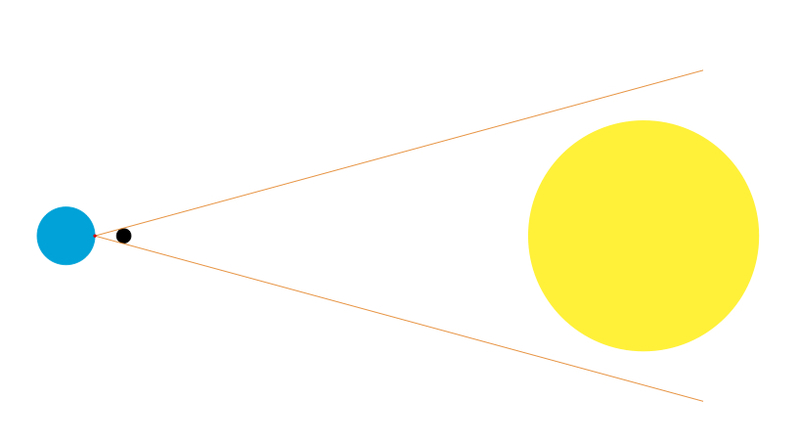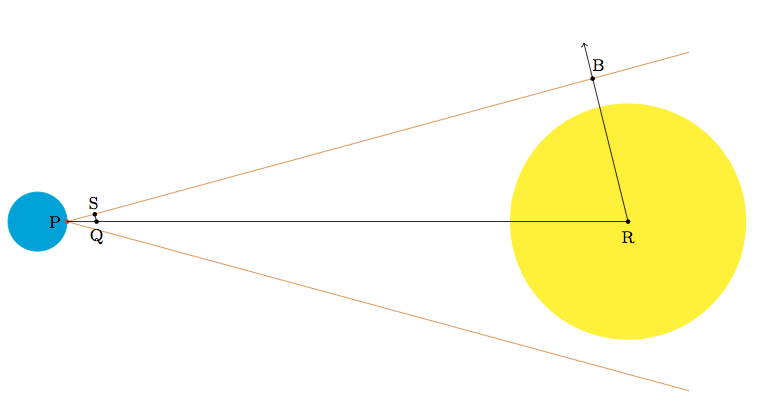# Solar Eclipse

Alignments to Content Standards: G-MG.A.1

A solar eclipse occurs when the moon passes between the sun and the earth. The eclipse is called partial if the moon only blocks part of the sun and total if the moon blocks out the entire sun. A picture (not drawn to scale) of the configuration of the earth, moon, and sun during a total eclipse at the point of the earth marked in red is shown below with the earth in blue, the moon black, and the sun yellow:The sun is approximately $93,000,000$ miles from the earth and the moon is about $239,000$ miles from the earth, with a range from about $225,600$ miles at its closest to $252,100$ at its furthest. The radius of the moon is approximately $1080$ miles.

1. Total eclipses are rare but do occur: given this information, how large could the radius of the sun be?
2. The radius of the sun is approximately $432,000$ miles. What is the furthest the moon can be from the earth for a total eclipse to occur? Does this help to explain why total eclipses are rare?

## IM Commentary

Total solar eclipses are quite rare, so much so that they make the news when they do occur. This task explores some of the reasons why. In addition to requiring the approrpiate configuration of the sun, moon, and earth, it turns out that the moon is only large enough to completely block out the sun when it is on the part of its orbit which brings it closest to the earth. Total eclipses are also very short in duration since the moon needs to be positioned nearly perfectly between the earth and the sun. Video showing a total solar eclipse can be seen below:

Solving the problem is a good application of similar triangles. If students have recently covered an abstract argument showing similiarty of triangles, as is needed here to show that $\triangle PSQ$ and $\triangle PBR$ are similar, then students might take this for granted and move to the interesting calculations which follow from this similarity.

The orbit of the earth around the sun is not circular and so the distance of 93,000,000 miles from the earth to the sun is an approximation. The teacher may wish to have students explore what happens when the sun is at its closest point and furthest point from the earth. Unlike the orbit of the moon, however, where the nearest and furthest points in its orbit differ by about $10 \%$, the nearest and furthest points in the earth's orbit of the sun only differ by about $3 \%$.

Due to the size and complexity of the numbers in this task, teachers may wish to supply calculators to ease this burden.

## Solution

1. For a total eclipse to occur, the sun must be completely within the two tangent lines from the point on the earth to the moon as shown in the picture. Suppose we call $P$ the point on the earth and we label the center of the moon $Q$ and the center of the sun $R$. These three points will be collinear when the moon is optimally placed to produce an eclipse at point $P$. Suppose $S$ is the point where the upper tangent line from $P$ to the moon meets the moon. This is all pictured below, with the moon removed from the picture in order to show triangle $PQS$ more clearly:Also pictured is ray $RB$, with $B$ on line $\overleftrightarrow{PS}$ chosen so that angles $PQS$ and $PRB$ are congruent. Angle $PSQ$ is a right angle because $\overleftrightarrow{PS}$ meets the circle representing the moon tangentially at $S$. Triangles $PSQ$ and $PBR$ share corresponding congruent angles $PQS$ and $PRB$ by hypothesis as well as angle $P$ which belongs to both triangles. Therefore angles $PSQ$ and $PBR$ are also congruent and angle $PBR$ is a right angle. By the AAA criterion for similarity, triangles $PSQ$ and $PBR$ are similar. Since corresponding sides of similar triangles have proportional side lengths this means that $$\frac{|RB|}{|PR|} = \frac{|QS|}{|PQ|}.$$

If $B$ is not on the sun or is on the boundary of the sun, then the moon will block all of the sun's rays. We know that $|PQ|$, the distance from the earth to the center of the moon is the distance of the earth to the moon plus the radius of the moon. At its smallest, this is about $226,680$ miles and at its largest about $253,180$ miles. We also know that $|PR|$ is the distance of the earth to the sun plus the radius of the sun. From the given information this is about $93,000,000 + r$ if $r$ denotes the radius of the sun. Since total eclipses do occur this tells us that $|RB| \geq r$ and the largest possible value of $|RB|$ would be $|RB| = r$. Plugging in what we know to the proportion at the end of the first paragraph gives $$\frac{r}{93,000,000 + r} = \frac{1080}{|PQ|}$$ and we know that $226,680 \leq |PQ| \leq 253,180$. Cross-multiplying and rearranging gives $$(|PQ| - 1080)r = 1080 \times 93,000,000$$ or $$r = \frac{1080 \times 93,000,000}{|PQ|-1080}.$$ The largest possible value of $r$ will come when $|PQ| - 1080$ is as small as possible, that is when the moon is as close to the earth as possible: this can be seen both from the structure of the expression for $r$ above or using geometric intuition as the closer the moon is to the earth the more of space it will obstruct. This corresponds to a value of $225,600$ miles for $|PQ| - 1080$. Plugging this into the above expression, the largest the radius of the sun could be while still allowing for a total eclipse is about $445,000$ miles.

Because the moon and sun have been modeled by circles, reflecting this picture about the line $\overleftrightarrow{PR}$ the same reasoning applies to rays emanating from the lower half of the sun being blocked (or not) by the lower half of the moon.

Note that the expression $$\frac{1080 \times 93,000,000}{225,600} \,\,\mbox{miles}.$$ for the maximum size of the sun, allowing for a total eclipse, makes intuitive sense: if the moon were twice as far away and twice as large it would ''appear'' identical. The fraction $\frac{93,000,000}{225,600}$ represents how many times as far away the sun is than the moon. So if it were this many times as large as the moon it would ''appear'' the same and therefore be perfectly blocked out by the moon (if correctly positioned). This plausible argument is made rigorous with the language of similar triangles used above.

2. We start with the equation from part (a) $$\frac{r}{93,000,000 + r} = \frac{1080}{|PQ|}.$$ We are given that the radius of the sun is about $432,000$ miles so this means that $r = 432,000$. Recall that this equation holds when the moon blocks out the sun exactly with no room to spare, that is when the point $B$ of the picture lies on the boundary of the sun. Solving for $|PQ|$ gives $$|PQ| = \frac{1080 \times (93,000,000 + 432,000)}{432,000} = 233,580.$$ This is much closer to 225,600 than to 252,100 and so it will only be during a very small period of the moon's orbit around the earth that it will be close enough to the earth to block out the entire sun. In addition, the relative position of earth, moon, and sun need to be in just the right order for a total eclipse to occur, so it is not suprising that these are so rare.